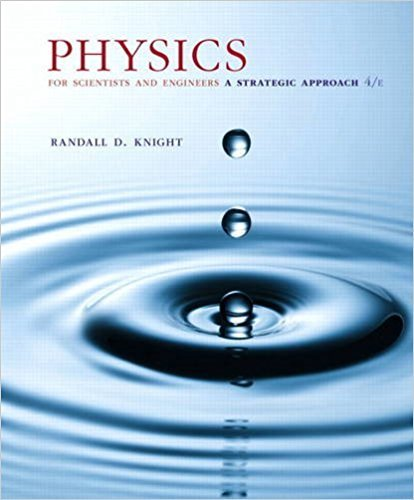×
×

# A rocket is fired from the earth to the moon at a speed ofISBN: 9780134081496 191

## Solution for problem 36.100 Chapter 36

Physics for Scientists and Engineers: A Strategic Approach, Standard Edition (Chs 1-36) | 4th Edition

• Textbook Solutions
• 2901 Step-by-step solutions solved by professors and subject experts
• Get 24/7 help from StudySoup virtual teaching assistantsPhysics for Scientists and Engineers: A Strategic Approach, Standard Edition (Chs 1-36) | 4th Edition

4 5 1 339 Reviews
26
1
Problem 36.100

A rocket is fired from the earth to the moon at a speed of 0.990c. Let two events be rocket leaves earth and rocket hits moon. a. In the earths reference frame, calculate x, t, and the spacetime interval s for these events. b. In the rockets reference frame, calculate x, t, and the spacetime interval s for these events. c. Repeat your calculations of part a if the rocket is replaced with a laser beam

Step-by-Step Solution:
Step 1 of 3
Step 2 of 3

Step 3 of 3

##### ISBN: 9780134081496

Since the solution to 36.100 from 36 chapter was answered, more than 283 students have viewed the full step-by-step answer. This textbook survival guide was created for the textbook: Physics for Scientists and Engineers: A Strategic Approach, Standard Edition (Chs 1-36), edition: 4. The full step-by-step solution to problem: 36.100 from chapter: 36 was answered by , our top Physics solution expert on 12/28/17, 08:06PM. This full solution covers the following key subjects: . This expansive textbook survival guide covers 42 chapters, and 4463 solutions. Physics for Scientists and Engineers: A Strategic Approach, Standard Edition (Chs 1-36) was written by and is associated to the ISBN: 9780134081496. The answer to “A rocket is fired from the earth to the moon at a speed of 0.990c. Let two events be rocket leaves earth and rocket hits moon. a. In the earths reference frame, calculate x, t, and the spacetime interval s for these events. b. In the rockets reference frame, calculate x, t, and the spacetime interval s for these events. c. Repeat your calculations of part a if the rocket is replaced with a laser beam” is broken down into a number of easy to follow steps, and 76 words.

Unlock Textbook Solution